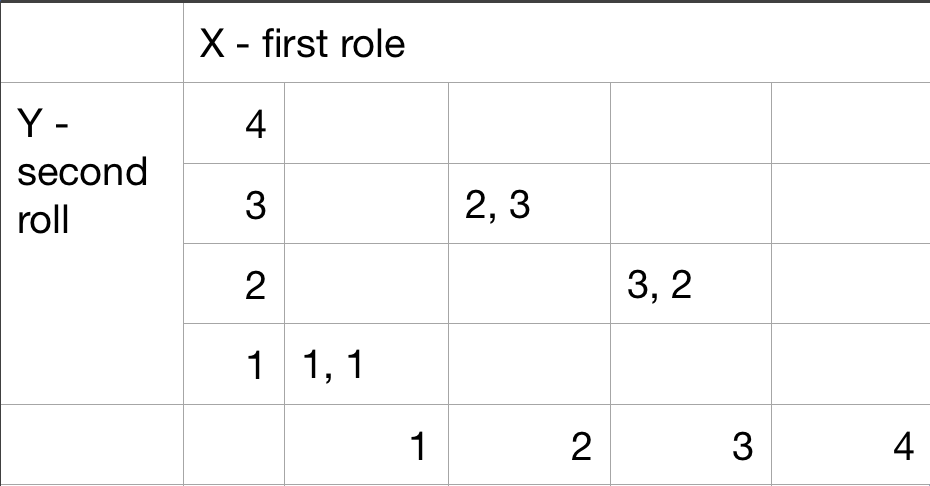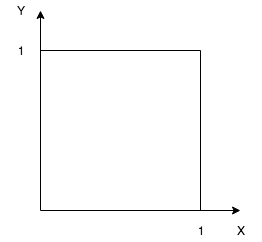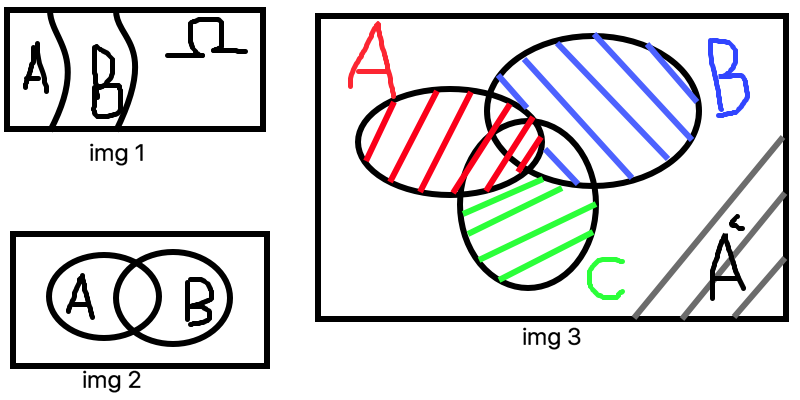# Probability Models and Axioms

## Sample space

• Describe possible outcomes
• Describe beliefs about likelihood outcomes

Possible outcomes:

• List (set) of possible outcomes, $$\Omega$$ (omega)
• List must be:
• Mutually exclusive
• Collectively exhaustive
• All the “right” granularity

Interpretation and uses of probabilities:

• Measure frequency of the event
• Description of beliefs / betting performance

### Sample space: discrete/finite exampleTable description

Sequential description

Two rolls of a tetrahedral die.

### Sample space: continuous example

$$(x, y)$$ such that $$0 \leqslant x, y \leqslant 1$$## Probability axioms

Event
is a subset of the sample space. Probability is assigned to eventsAxioms:

• Nonnegativity: $$P(A) \geqslant 0$$
• Normalization: $$P(\Omega) = 1$$
• (Finite) Additivity: if $$A \cap B = \varnothing$$, then $$P(A \cup B) = P(A) + P(B)$$

Some simple consequences of the axioms:

• $$P(\varnothing) = 0$$
• $$P(A) + P(A^c) = 1$$
• if $$A_1, …, A_k$$ disjoint, then $$P(A \cup … \cup A_k) = \sum_{i=1}^{k}P(A_i)$$
• $$P(\{S_1, S_2, …, S_k\}) = P(\{S_1\} \cup \{S_2\} \cup … \cup \{S_k\}) = P(\{S_1\}) + … P(\{S_k\}) = P(S_1) + … + P(S_k)$$

More consequences of the axioms:

• if $$A \subset B$$, then $$P(A) \leqslant P(B)$$ (img 1)
• $$P(A \cup B) = P(A) + P(B) - P(A \cap B) = P(A) + P(A^c \cap B)$$ (img 2)
• $$P(A \cup B) \leqslant P(A) + P(B)$$ - union bound
• $$P(A \cup B \cup C) = P(A) + P(A^c \cap B) + P(A^c \cap B^c \cap C)$$ (img 3)## Probability calculations

### Discrete finite example

Discrete uniform law:

• Assume $$\Omega$$ finite consist of $$n$$ equally likely elements
• Assume $$A$$ consist of $$k$$ elements, then

$$P(A) = k * frac{1}{n}$$

every outcome prob == $$\frac{1}{16}$$

• $$P(X = 1) = 4 * \frac{1}{16}$$
• let $$Z = min(X, Y)$$
• $$P(Z = 4) = 1/16$$
• $$P(Z = 2) = 5 * \frac{1}{16}$$

### Discrete continuous example

Suppose we have $$(x, y)$$ such that $$0 \leq x, y, \leq 1$$

Uniform probability law: Probability = Area

$$P(\{(x, y) | x + y \leq 1/2 \})$$ = triangle area = $$\frac{1}{2} * \frac{1}{2} * \frac{1}{2}$$

$$P(\{0.5, 0.3 \})$$ = area of one point = $$0$$

### Probability calculations step

• Specify sample space
• Specify a probability law
• Identify an event of interest(if possible - graphical way)
• Calculate …

Let’s we have sample space $$\{1, 2, 3, … \}$$

$$P(n) = \frac{1}{2^n}, n=1, 2..$$

$$\sum_{n=1}^{\infty} \frac{1}{2^n} = \frac{1}{2} \sum_{n=0}^{\infty} \frac{1}{2^n} = \frac{1}{2} * \frac{1}{1 - 1/2} = 1$$

P(outcome is even) = $$P(\{2, 4, 6, … \} ) = P(\{2\} \cup \{4\} \cup ..) = P(2) + P(4) + .. =$$

$$= \frac{1}{2^2} + \frac{1}{2^4} + … = \frac{1}{4}(1 + \frac{1}{4} + \frac{1}{4^2} + …) = \frac{1}{4} * \frac{1}{1 - 1/4} = \frac{1}{3}$$

### Countable additivity axiom

Strengthens the finite additivity axiom:

• if $$A_1, A_2. A_3$$ is an finite sequence of disjoint events, then $$P(A_1 \cup A_2 \cup A_3 …) = P(A_1) + P(A_2) + P(A_3)$$
• additivity holds only for “countable” sequence of events
• the unit square(similarly the real line, etc.) is not countable. (It’s elements cannot be arranged in a sequence)
• Area” is a legitimate probability law on the unit square, as long as we do not try to assign probabilities/areas to “very strange” sets